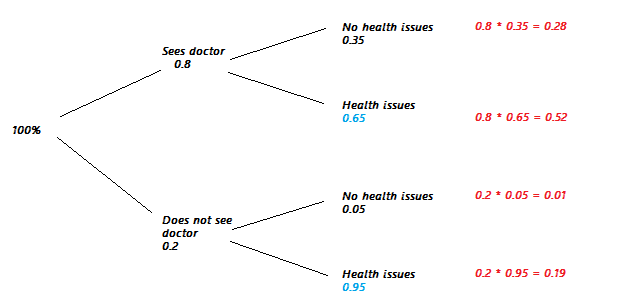# Law of Total Probability

## What is the Law of Total Probability?

The Law of Total Probability is a concept within probability theory that is used to describe the total probability of an outcome. The law is defined as the total probability that event A, with its associated probabilities, will happen given the events B, with their associated probabilities.

## How does the Law of Total Probability work?

It is helpful to understand this concept with an example. Imagine there are two different car manufacturers. Company A makes cars that go 200,000 miles 90% of the time, and Company B makes cars that go 200,000 miles 99% of the time. When renting a car, you know there's a 40% chance of getting a car from Company A, and a 60% chance of getting a car from Company B. Given the probabilities, what is the chance that a car you rent will be able to go 200,000 miles?

This problem can be solved by using the Law of Total Probability. We can calculate the probability by multiplying the probability of getting car A with the probability of it driving 200,00 miles, and adding that the the probability of getting car B multiplied by the probability of that car driving 200,000 miles. Pictured below is an image that conceptualizes this problem, but with different variables.Source